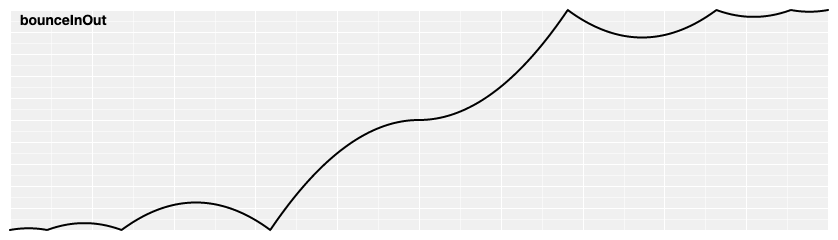d3-ease

Easing is a method of distorting time to control apparent motion in animation. It is most commonly used for slow-in, slow-out. By easing time, animated transitions are smoother and exhibit more plausible motion.

The easing types in this module implement the ease method, which takes a normalized time t and returns the corresponding “eased” time . Both the normalized time and the eased time are typically in the range [0,1], where 0 represents the start of the animation and 1 represents the end; some easing types, such as elastic, may return eased times slightly outside this range. A good easing type should return 0 if t = 0 and 1 if t = 1. See the easing explorer for a visual demonstration.

These easing types are largely based on work by Robert Penner.

Installing

If you use NPM, `npm install d3-ease`. Otherwise, download the latest release. You can also load directly from d3js.org, either as a standalone library or as part of D3 4.0. AMD, CommonJS, and vanilla environments are supported. In vanilla, a `d3` global is exported:

``````<script src="https://d3js.org/d3-ease.v1.min.js"></script>
<script>

var ease = d3.easeCubic;

</script>
``````

API Reference

# ease(t)

Given the specified normalized time t, typically in the range [0,1], returns the “eased” time , also typically in [0,1]. 0 represents the start of the animation and 1 represents the end. A good implementation returns 0 if t = 0 and 1 if t = 1. See the easing explorer for a visual demonstration. For example, to apply cubic easing:

``````var te = d3.easeCubic(t);
``````

Similarly, to apply custom elastic easing:

``````// Before the animation starts, create your easing function.
var customElastic = d3.easeElastic.period(0.4);

// During the animation, apply the easing function.
var te = customElastic(t);
``````

# d3.easeLinear(t) <>

Linear easing; the identity function; linear(t) returns t.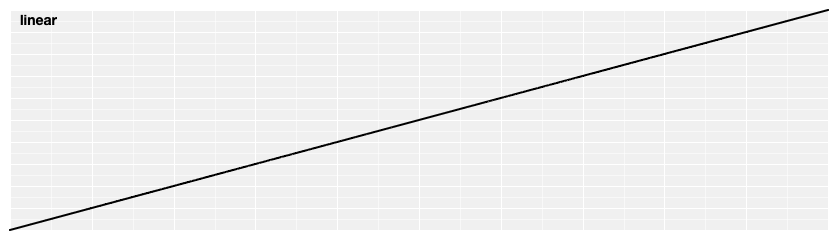# d3.easePolyIn(t) <>

Polynomial easing; raises t to the specified exponent. If the exponent is not specified, it defaults to 3, equivalent to cubicIn.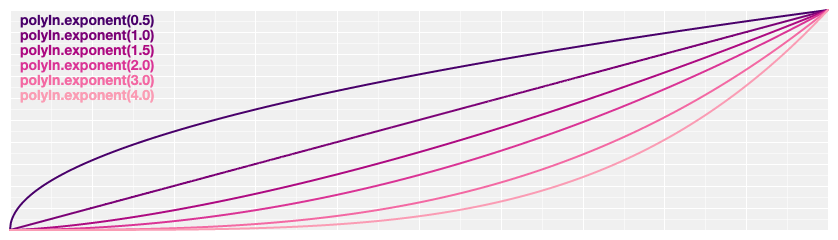# d3.easePolyOut(t) <>

Reverse polynomial easing; equivalent to 1 - polyIn(1 - t). If the exponent is not specified, it defaults to 3, equivalent to cubicOut.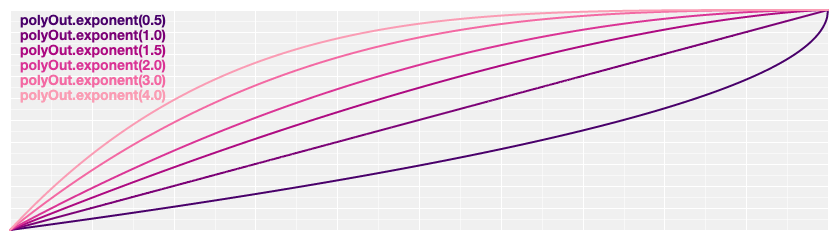# d3.easePoly(t) <>
# d3.easePolyInOut(t) <>

Symmetric polynomial easing; scales polyIn for t in [0, 0.5] and polyOut for t in [0.5, 1]. If the exponent is not specified, it defaults to 3, equivalent to cubic.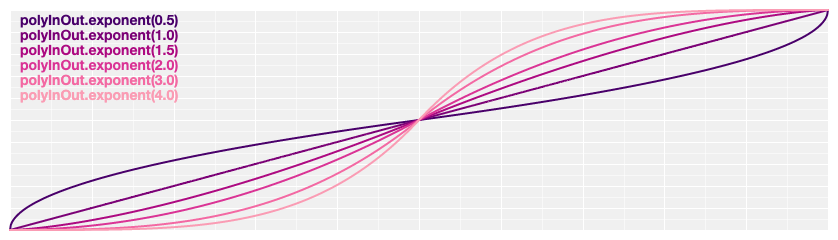# poly.exponent(e) <>

Returns a new polynomial easing with the specified exponent e. For example, to create equivalents of linear, quad, and cubic:

``````var linear = d3.easePoly.exponent(1),
cubic = d3.easePoly.exponent(3);
``````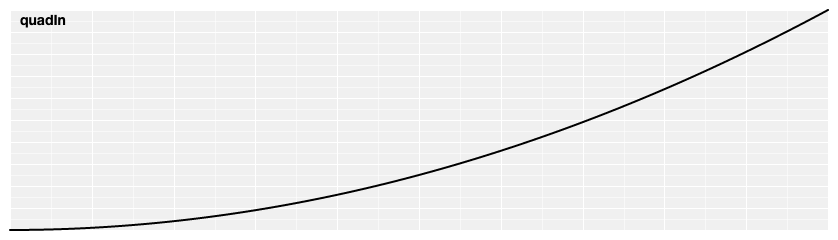Reverse quadratic easing; equivalent to 1 - quadIn(1 - t). Also equivalent to polyOut.exponent(2).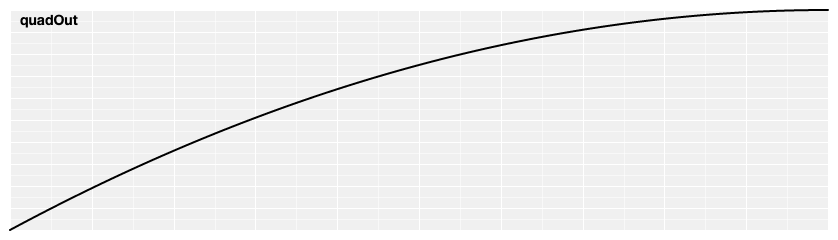Symmetric quadratic easing; scales quadIn for t in [0, 0.5] and quadOut for t in [0.5, 1]. Also equivalent to poly.exponent(2).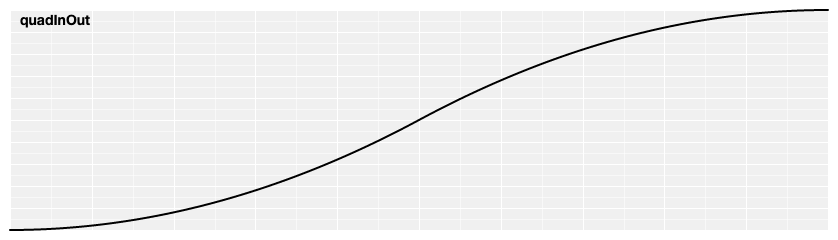# d3.easeCubicIn(t) <>

Cubic easing; equivalent to polyIn.exponent(3).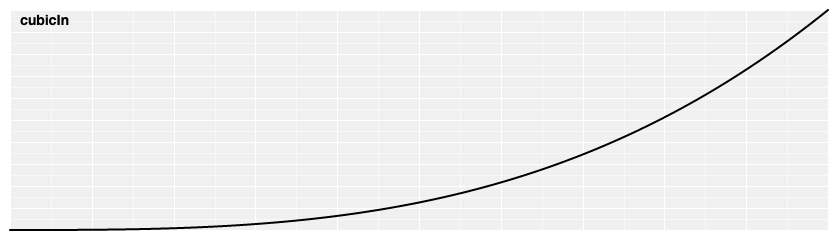# d3.easeCubicOut(t) <>

Reverse cubic easing; equivalent to 1 - cubicIn(1 - t). Also equivalent to polyOut.exponent(3).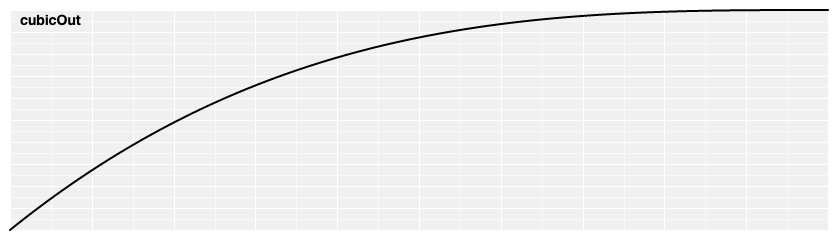# d3.easeCubic(t) <>
# d3.easeCubicInOut(t) <>

Symmetric cubic easing; scales cubicIn for t in [0, 0.5] and cubicOut for t in [0.5, 1]. Also equivalent to poly.exponent(3).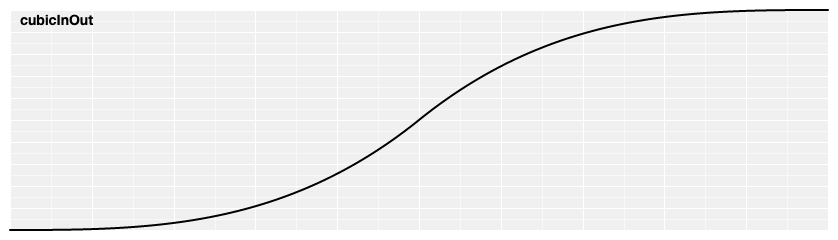# d3.easeSinIn(t) <>

Sinusoidal easing; returns sin(t).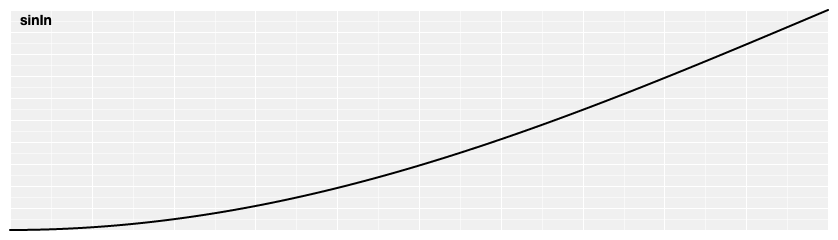# d3.easeSinOut(t) <>

Reverse sinusoidal easing; equivalent to 1 - sinIn(1 - t).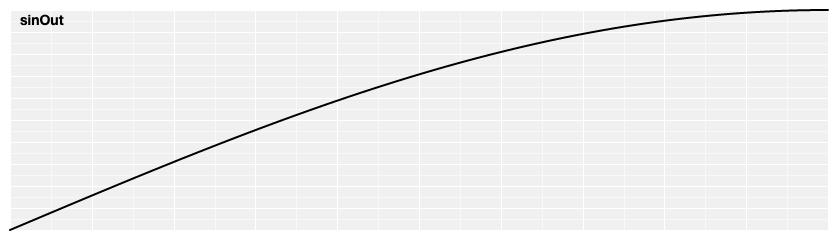# d3.easeSin(t) <>
# d3.easeSinInOut(t) <>

Symmetric sinusoidal easing; scales sinIn for t in [0, 0.5] and sinOut for t in [0.5, 1].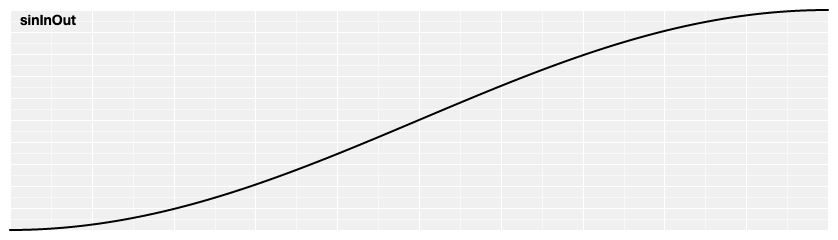# d3.easeExpIn(t) <>

Exponential easing; raises 2 to the exponent 10 * (t - 1).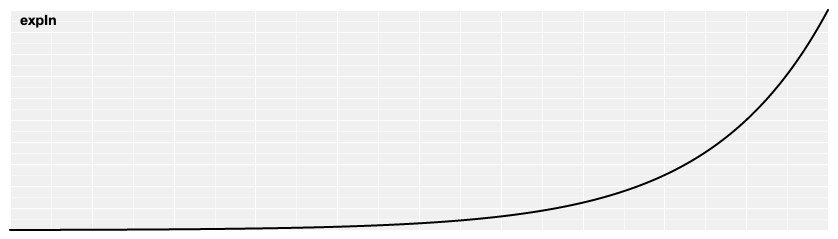# d3.easeExpOut(t) <>

Reverse exponential easing; equivalent to 1 - expIn(1 - t).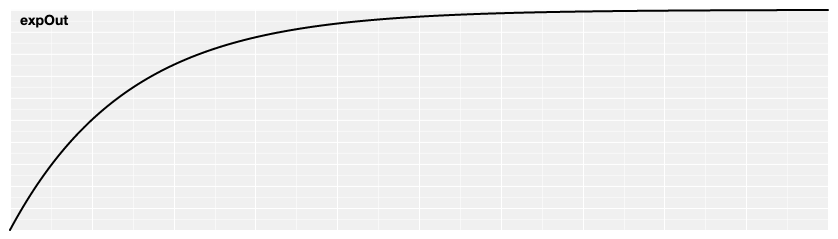# d3.easeExp(t) <>
# d3.easeExpInOut(t) <>

Symmetric exponential easing; scales expIn for t in [0, 0.5] and expOut for t in [0.5, 1].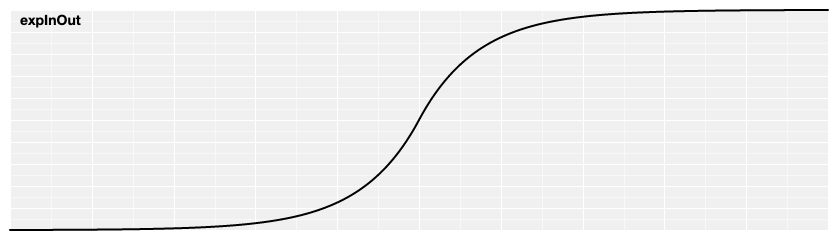# d3.easeCircleIn(t) <>

Circular easing.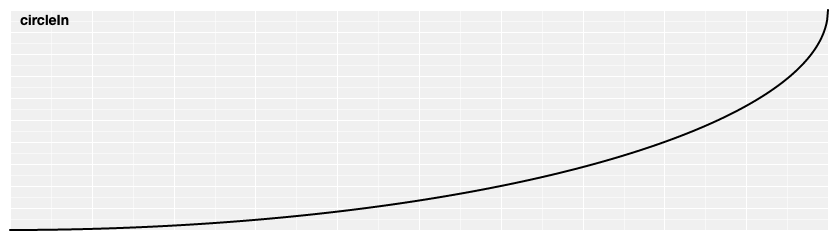# d3.easeCircleOut(t) <>

Reverse circular easing; equivalent to 1 - circleIn(1 - t).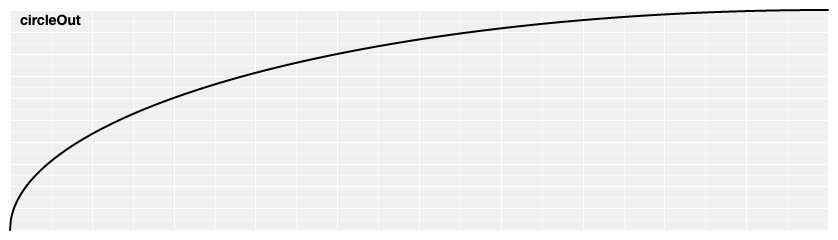# d3.easeCircle(t) <>
# d3.easeCircleInOut(t) <>

Symmetric circular easing; scales circleIn for t in [0, 0.5] and circleOut for t in [0.5, 1].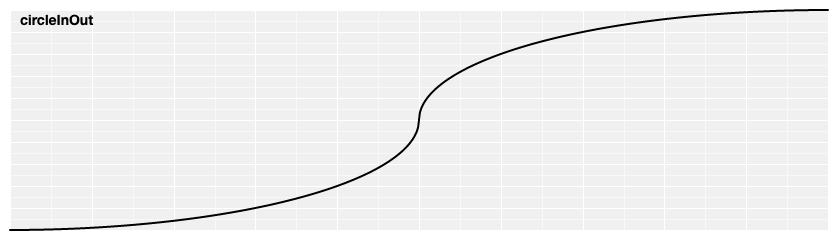# d3.easeElasticIn(t) <>

Elastic easing, like a rubber band. The amplitude and period of the oscillation are configurable; if not specified, they default to 1 and 0.3, respectively.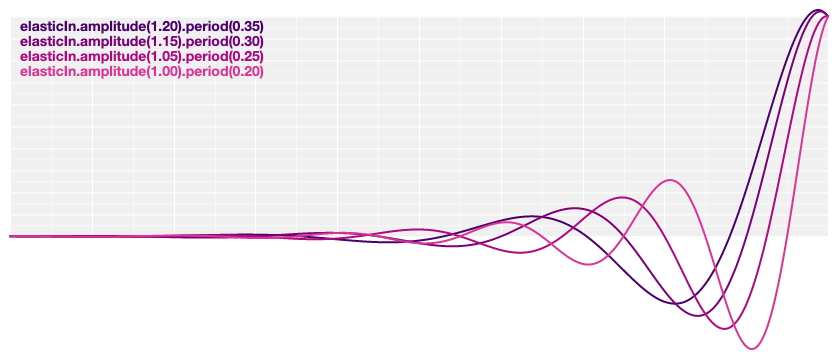# d3.easeElastic(t) <>
# d3.easeElasticOut(t) <>

Reverse elastic easing; equivalent to 1 - elasticIn(1 - t).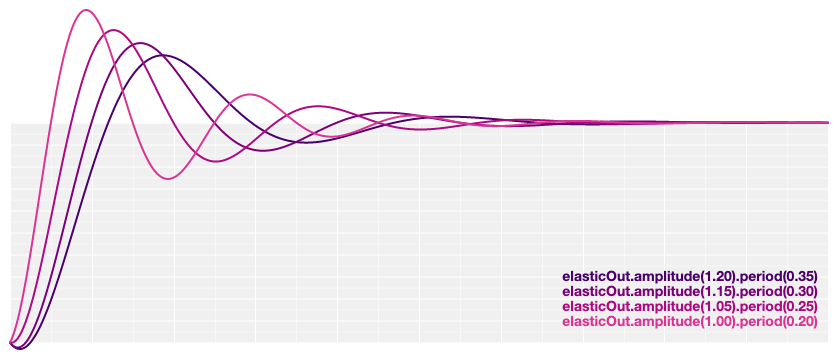# d3.easeElasticInOut(t) <>

Symmetric elastic easing; scales elasticIn for t in [0, 0.5] and elasticOut for t in [0.5, 1].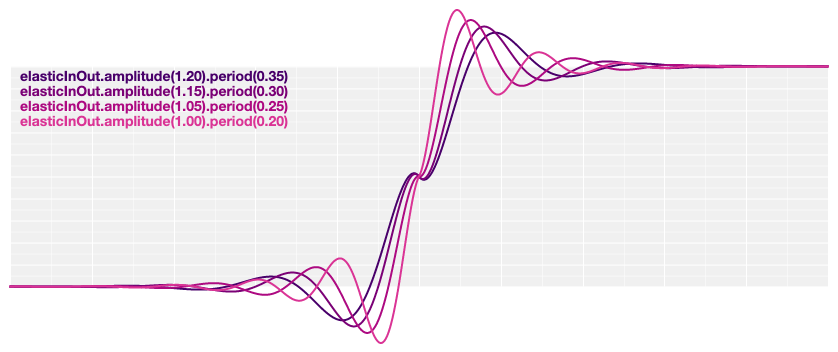# elastic.amplitude(a) <>

Returns a new elastic easing with the specified amplitude a.

# elastic.period(p) <>

Returns a new elastic easing with the specified period p.

# d3.easeBackIn(t) <>

Anticipatory easing, like a dancer bending his knees before jumping off the floor. The degree of overshoot is configurable; it not specified, it defaults to 1.70158.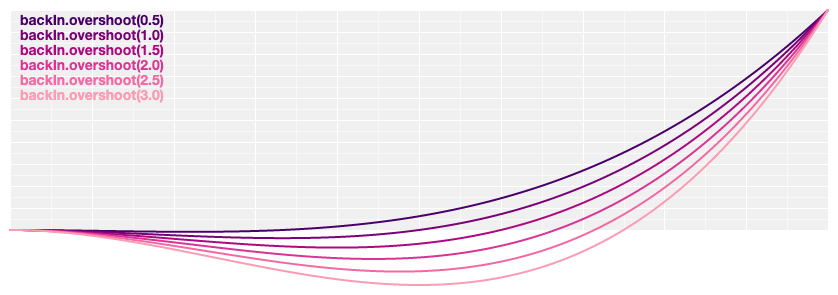# d3.easeBackOut(t) <>

Reverse anticipatory easing; equivalent to 1 - backIn(1 - t).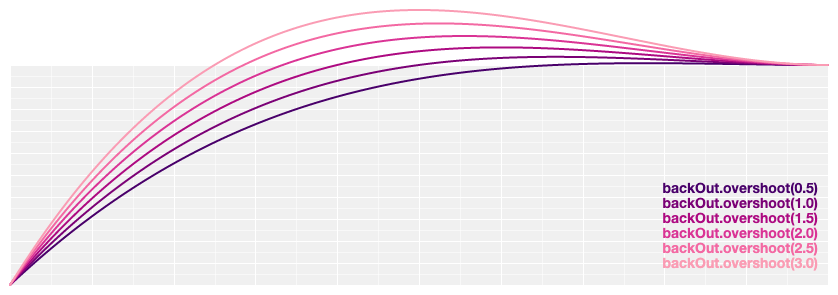# d3.easeBack(t) <>
# d3.easeBackInOut(t) <>

Symmetric anticipatory easing; scales backIn for t in [0, 0.5] and backOut for t in [0.5, 1].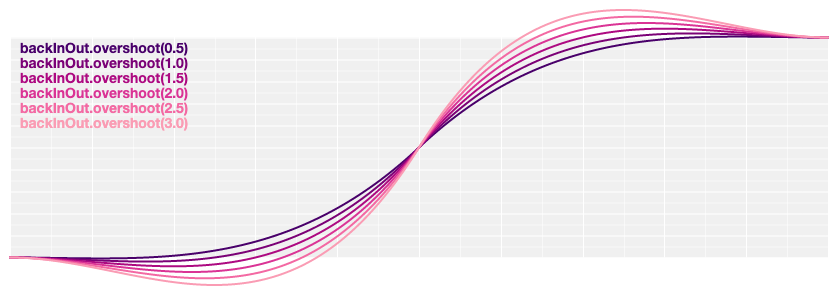# back.overshoot(s) <>

Returns a new back easing with the specified overshoot s.

# d3.easeBounceIn(t) <>

Bounce easing, like a rubber ball.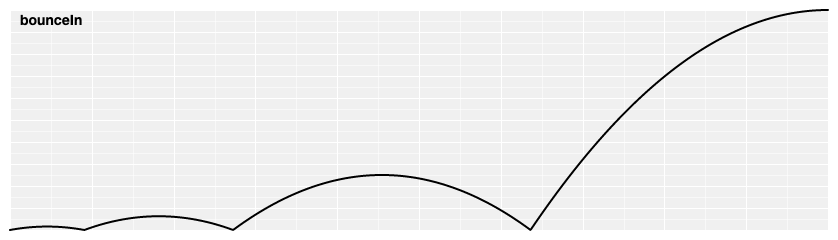# d3.easeBounce(t) <>
# d3.easeBounceOut(t) <>

Reverse bounce easing; equivalent to 1 - bounceIn(1 - t).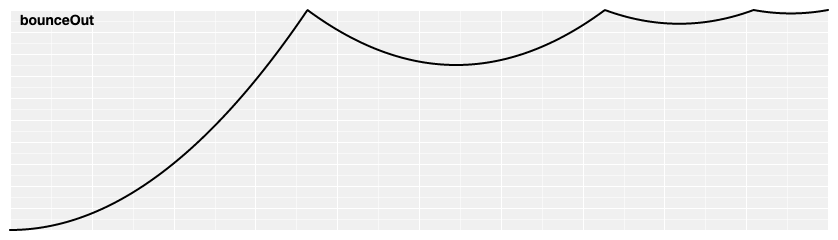# d3.easeBounceInOut(t) <>

Symmetric bounce easing; scales bounceIn for t in [0, 0.5] and bounceOut for t in [0.5, 1].